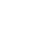1)      The average velocity for an interval can be found by finding the slope of that interval. Using the tangent method or mid-point method to find the rise/run will give you the average velocity of the cart at that interval.

2)      For constant acceleration, the average velocity for the interval will be equal to the instantaneous velocity at the half-time of the interval.

3)      The average velocity for an interval on a velocity- time graph must be plotted on one specific point on the line to represent instantaneous velocity.

4)      If the acceleration is constant, then the velocity-time graph will be a linear line. However if the acceleration deviates positively then the velocity-time graph will gain a curved or parabolic shape.

5)      Based of the data from the velocity time graph, the cart is experiencing non-uniform motion.

6)      The average acceleration of the cart is 4.1m/s² or 4.1cm/s².

7)      The area underneath the each interval was the relatively the same as the displacement of the cart at that interval. It should however be exactly the same as the displacement of the cart at the interval. The values may be different simply due to rounding during the calculations.9)

a) To create a velocity-time graph from a position – time graph divide the displacement of each interval by the time at of each interval; the resultant will be the average velocity of each interval (Or use the slope). Then plot that average velocity as the dependent variable on a velocity-time graph; plot time as the independent variable.

b) To create a position- time graph from a velocity- time graph find the average velocity of each interval. This can be done by finding the area underneath the line at each specific interval and then plotting each value in accordance with its time interval on a position-time graph.

c) To create an acceleration- time graph from a velocity time graph find the slope of each interval. Once the average acceleration (slope) of each interval is calculated, plot each average acceleration value with its appropriate time interval.

d) To create a velocity- time graph from an acceleration- time graph find the average acceleration of each time interval (This can be done by using the area underneath the curve). Once that is done plot it with its corresponding time interval.

10) The ticker tape cart experience uniform acceleration and had a constant magnitude 4.1m/s² [fwd] down the ramp.

In conclusion, the velocity time graph of the cart down the ramp had a relative, if not completely linear shape. To determine the displacement of the cart, find the area underneath the line of the velocity-time graph and to find the acceleration of the cart, find the slope of the line of the velocity-time graph. The data collected from this experiment was quite accurate and testing was performed quite smoothly, however as stated early, there is always room for improvement.

Cite this article as: William Anderson (Schoolworkhelper Editorial Team), "Ticker Tap Lab Discussion Questions," in SchoolWorkHelper, 2019, https://schoolworkhelper.net/ticker-tap-lab-discussion-questions/.#### -We are looking for previous essays, labs and assignments that you aced!

-We will review and post them on our website.
-Ad revenue is used to support children in developing nations.
-We help pay for cleft palate repair surgeries through Operation Smile and Smile Train.1 Comment
Inline Feedbacks